Exterior Angles in a Triangle MathBitsNotebook.com Terms of Use   Contact Person: Donna Roberts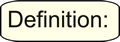An exterior angle of a triangle is an angle formed by one side of the triangle and the extension of an adjacent side of the triangle.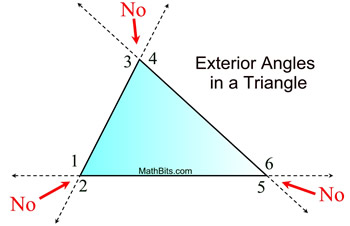FACTS: • Every triangle has 6 exterior angles, two at each vertex. • Angles 1 through 6 are exterior angles. • Notice that the "outside" angles that are "vertical" to the angles inside the triangle are NOT called exterior angles of a triangle.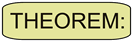The measure of an exterior angle of a triangle is equal to the sum of the measures of the two non-adjacent interior angles. (Non-adjacent interior angles may also be referred to as remote interior angles.)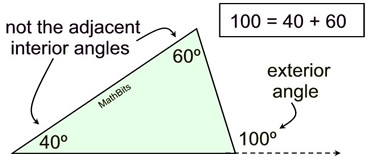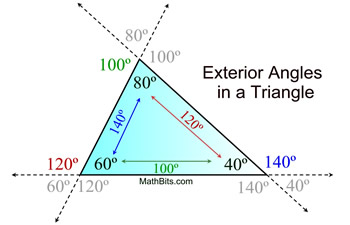FACTS: • An exterior ∠ is equal to the addition of the two Δ angles not right next to it. 140º = 60º + 80º;        120º = 80º + 40º; 100º = 60º + 40º • An exterior angle is supplementary to its adjacent Δ angle. 140º is supp to 40º • The 2 exterior angles at each vertex are = in measure because they are vertical angles. • The exterior angles (taken one at a vertex) always total 360º

 Examples:

 1.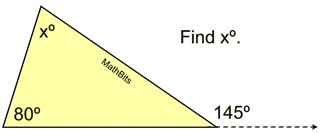Solution: Using the Exterior Angle Theorem
145 = 80 + x
x = 65

Now, if you forget the Exterior Angle Theorem, you can still get the answer by noticing that a straight angle has been formed at the vertex of the 145º angle. See Example 2.
 2.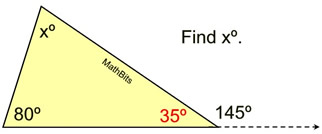Solution: I forgot the Exterior Angle Theorem.
The angle adjacent to 145º will form a straight angle along with 145º adding to 180º. That angle is 35º.
Now use rule that sum of ∠s in Δ = 180º.
35 + 80 + x = 180
115 + x = 180
x = 65
 3.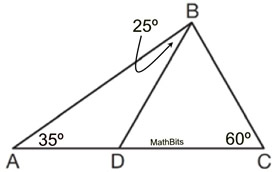Find m∠DBC.
Solution:
∠BDC is an exterior angle for ΔABD.
m∠BDC = 35 + 25
m∠BDC = 60º
180 = m∠DBC + 60 + 60

m∠DBC = 60º
4.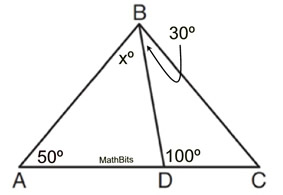Find xº.
Solution:
100 = x + 50
x = 50º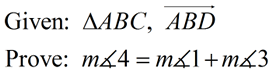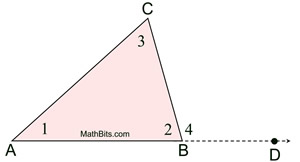Statements Reasons 1.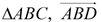1. Given 2. ∠2 and ∠4 form a linear pair 2. A linear pair is 2 adjacent ∠s whose non-common sides form opposite rays. 3. ∠2 supp ∠4 3. If 2∠s form a linear pair, they are supplementary. 4.  m∠2 + m∠4 = 180 4. Supplementary ∠s are 2 ∠s the sum of whose measures is 180. 5.  m∠1 + m∠2 + m∠3 = 180 5. The measures of the angles of a triangle add to 180º. 6.  m∠2 + m∠4 = m∠1 + m∠2 + m∠3 6. Substitution 7.  m∠2 = m∠2 7. Reflexive Property (or quantity is = itself) 8.  m∠4 = m∠1 + m∠3 8. Subtraction of Equalities